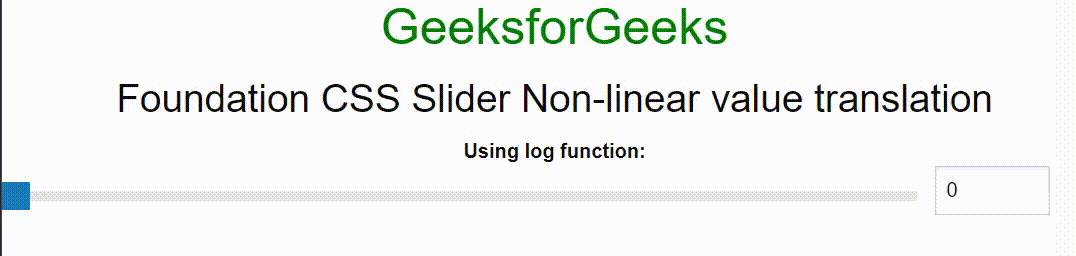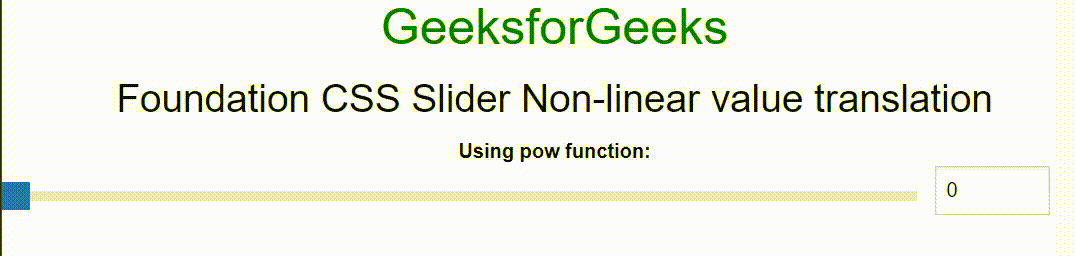Open in App
Not now

# Foundation CSS Slider Non-linear value translation

• Last Updated : 10 Mar, 2022

Foundation CSS is an open-source and responsive front-end framework created by ZURB in September 2011 that makes it simple to create stunning responsive websites, apps, and emails that operate on any device. Many companies, like Facebook, eBay, Mozilla, Adobe, and even Disney, use it. This framework is based on bootstrap, which is similar to SaaS. It’s more complex, versatile, and configurable. It also comes with a command-line interface, making it simple to use with module bundlers. Email framework provides you with responsive HTML emails, which can be read on any device. Foundation for Apps allows you to build fully responsive web applications.

The Slider is used to specify the range of values when we drag the slider. We can create the slider to set the range and users can choose any value by dragging the slider. Slider Non-linear value translation is used to focus the important values on the slider. When the slider focuses on the higher values then we use the log type position value function and when the slider focus on the lower values then we use the pow type position value function. In this article, we will discuss the Slider Non-linear value translation in Foundation CSS.

Foundation CSS Slider Non-linear value translation Attribute:

• data-position-value-function: It is used to focus the important values on the slider. When we assign a log function to it then it will focus on higher values and when we assign the pow function then lower values will be focused.

Syntax:

```<div class="slider"
data-slider
data-position-value-function="VALUE" >
<span class="slider-handle" data-slider-handle
role="slider" tabindex="1" aria-controls="">
</span>
</div>```

Example 1: The following code demonstrates the Slider Non-linear value translation using the log function.

## HTML

 ` ` `<``html``> ` ` `  `<``head``> ` `    ``` `    ``<``link` `rel``=``"stylesheet"`  `          ``href``= ` `"https://cdn.jsdelivr.net/npm/foundation-sites@6.7.4/dist/css/foundation.min.css"` `/> ` `    ``` `    ``<``script` `src``= ` `"https://cdnjs.cloudflare.com/ajax/libs/foundation/6.0.1/js/vendor/jquery.min.js"``> ` `    `` ` `    ``<``script` `src``= ` `"https://cdn.jsdelivr.net/npm/foundation-sites@6.5.1/dist/js/foundation.min.js"``> ` `    `` ` ` ` ` `  `<``body``> ` `    ``<``center``> ` `        ``<``h2` `style``=``"color: green"``> ` `            ``GeeksforGeeks ` `        `` ` `        ``<``h3``> ` `            ``Foundation CSS Slider Non-linear value translation ` `        `` ` `        ``<``strong``> ` `            ``Using log function: ` `        `` ` ` `  `        ``<``div` `class``=``"grid-x grid-margin-x"``> ` ` `  `            ``<``div` `class``=``"cell small-10"``> ` `                ``<``div` `class``=``"slider"`  `                     ``data-slider ` `                     ``data-position-value-function``=``"log"``> ` ` `  `                    ``<``span` `class``=``"slider-handle"`  `                          ``data-slider-handle  ` `                          ``role``=``"slider"` `                          ``tabindex``=``"1"` `                          ``aria-controls``=``"slider-value"``> ` `                    `` ` `                `` ` `            `` ` `            ``<``div``> ` `                ``<``input` `type``=``"number"` `id``=``"slider-value"``> ` `            `` ` `        `` ` `    `` ` `    ``<``script``> ` `        ``\$(document).ready(function () { ` `            ``\$(document).foundation(); ` `        ``}); ` `    `` ` ` ` ` `  ``

Output:Foundation CSS Slider Non-linear value translation

Example 2: The following code demonstrates the Slider Non-linear value translation using the pow function.

## HTML

 ` ` `<``html``> ` ` `  `<``head``> ` `    ``` `    ``<``link` `rel``=``"stylesheet"` `          ``href``= ` `"https://cdn.jsdelivr.net/npm/foundation-sites@6.7.4/dist/css/foundation.min.css"` `/> ` `    ``` `    ``<``script` `src``= ` `"https://cdnjs.cloudflare.com/ajax/libs/foundation/6.0.1/js/vendor/jquery.min.js"``> ` `    `` ` `    ``<``script` `src``= ` `"https://cdn.jsdelivr.net/npm/foundation-sites@6.5.1/dist/js/foundation.min.js"``> ` `    `` ` ` ` ` `  `<``body``> ` `    ``<``center``> ` `        ``<``h2` `style``=``"color: green"``> ` `            ``GeeksforGeeks ` `        `` ` `        ``<``h3``> ` `            ``Foundation CSS Slider Non-linear value translation ` `        `` ` `        ``<``strong``> ` `            ``Using pow function: ` `        `` ` `        ``<``div` `class``=``"grid-x grid-margin-x"``> ` `            ``<``div` `class``=``"cell small-10"``> ` `                ``<``div` `class``=``"slider"` `                     ``data-slider ` `                     ``data-position-value-function``=``"pow"``> ` ` `  `                    ``<``span` `class``=``"slider-handle"`  `                          ``data-slider-handle  ` `                          ``role``=``"slider"` `                          ``tabindex``=``"1"` `                          ``aria-controls``=``"slider-value"``> ` `                    `` ` `                `` ` `            `` ` `            ``<``div``> ` `                ``<``input` `type``=``"number"`  `                       ``id``=``"slider-value"``> ` `            `` ` `        `` ` `    `` ` `    ``<``script``> ` `        ``\$(document).ready(function () { ` `            ``\$(document).foundation(); ` `        ``}); ` `    `` ` ` ` ` `  ``

Output:Foundation CSS Slider Non-linear value translation

My Personal Notes arrow_drop_up
Related Articles